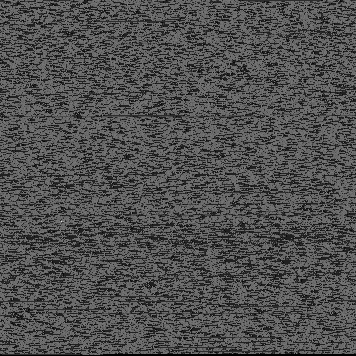﻿ JS压缩的另类方法 -- 将JS文件压缩成PNG图像存储方法 - Javascript - 郑州网建

# JS压缩的另类方法 -- 将JS文件压缩成PNG图像存储方法

YUI压缩工具 (http://developer.yahoo.com/yui/compressor/)
Dean Edwards Packer (http://dean.edwards.name/packer/)
JSMin (http://crockford.com/JavaScript/jsmin)
UglifyJS (http://marijnhaverbeke.nl/uglifyjs)`\$filename = "prototype-1.6.0.2.js";  if (file_exists(\$filename)) {      \$iFileSize = filesize(\$filename);      \$iWidth = ceil(sqrt(\$iFileSize / 1));      \$iHeight = \$iWidth;      \$im = imagecreatetruecolor(\$iWidth, \$iHeight);      \$fs = fopen(\$filename, "r");      \$data = fread(\$fs, \$iFileSize);      fclose(\$fs);      \$i = 0;      for (\$y=0;\$y<\$iHeight;\$y++) {          for (\$x=0;\$x<\$iWidth;\$x++) {              \$ord = ord(\$data[\$i]);              imagesetpixel(\$im,                   \$x, \$y,                  imagecolorallocate(\$im,                      \$ord,                      \$ord,                      \$ord                 )              );              \$i++;          }      }      header("Content-Type: image/png");      imagepng(\$im);      imagedestroy(\$im);  }  ?> `

`function loadPNGData(strFilename, fncCallback) {      // test for canvas and getImageData      var bCanvas = false;      var oCanvas = document.createElement("canvas");      if (oCanvas.getContext) {          var oCtx = oCanvas.getContext("2d");          if (oCtx.getImageData) {              bCanvas = true;          }      }      if (bCanvas) {          var oImg = new Image();          oImg.style.position = "absolute";          oImg.style.left = "-10000px";          document.body.appendChild(oImg);          oImg.onload = function() {              var iWidth = this.offsetWidth;              var iHeight = this.offsetHeight;              oCanvas.width = iWidth;              oCanvas.height = iHeight;              oCanvas.style.width = iWidth+"px";              oCanvas.style.height = iHeight+"px";              var oText = document.getElementById("output");              oCtx.drawImage(this,0,0);              var oData = oCtx.getImageData(0,0,iWidth,iHeight).data;              var a = [];              var len = oData.length;              var p = -1;              for (var i=0;i`